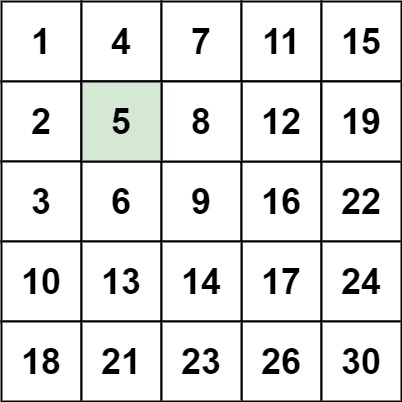# 若只如初见

0%

## 问题描述

Write an efficient algorithm that searches for a target value in an m x n integer matrix. The matrix has the following properties:

Integers in each row are sorted in ascending from left to right.
Integers in each column are sorted in ascending from top to bottom.• m == matrix.length
• n == matrix[i].length
• 1 <= n, m <= 300
• -109 <= matix[i][j] <= 109
• All the integers in each row are sorted in ascending order.
• All the integers in each column are sorted in ascending order.
• -109 <= target <= 109

## 解题思路

• 行列均为增长的顺序，又是搜索target，逐行采用二分法。

• JavaScript

• 时间复杂度：O(mlogn)
• 空间复杂度：O(1)